### Day 56 - Simplifying Radicals - 04.08.15

• Unit 5 Test on Friday, April 17th!

Questions

Bell Ringer
1. Simplify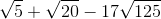.

1.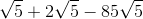2.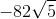3.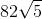4.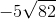5. none of the above

2. Simplify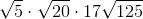.

1.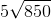2.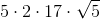3.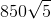4.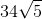5. none of the above

3. Simplify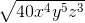.

1.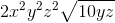2.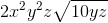3.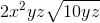4.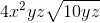5. none of the above

4. Simplify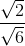.

1.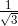2.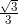3.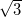4.5. none of the above

5. Simplify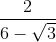.

1.2.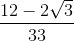3.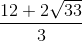4.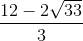5. none of the above

Review
• Square Roots and Rational Exponents

Lesson

Exit Ticket
• Posted on the board at the end of the block!
Lesson Objective(s)
• How can radicals be simplified?
Skills
1. Explain why the sum of two square roots is not equal to the square root of their sum.
2. Convert expressions to simplest radical form.

#### In-Class Help Requests

Standard(s)
• CC.9-12.A.REI.2 Understand solving equations as a process of reasoning and explain the reasoning. Solve simple rational and radical equations in one variable, and give examples showing how extraneous solutions may arise.
• CC.9-12.N.RN.1 Extend the properties of exponents to rational exponents. Explain how the definition of the meaning of rational exponents follows from extending the properties of integer exponents to those values, allowing for a notation for radicals in terms of rational exponents. For example, we define 5^(1/3) to be the cube root of 5 because we want [5^(1/3)]^3 = 5^[(1/3) x 3] to hold, so [5^(1/3)]^3 must equal 5.
• CC.9-12.N.RN.2 Extend the properties of exponents to rational exponents. Rewrite expressions involving radicals and rational exponents using the properties of exponents.

Mathematical Practice(s)
• #1 - Make sense of problems and persevere in solving them
• #2 - Reason abstractly and quantitatively
• #7 - Look for and make use of structure

Past Checkpoints
• N/A